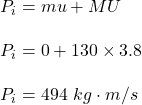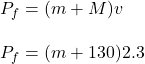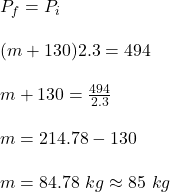During a football game, a receiver has just caught a pass and is standing still. Before he can move, a tackler, running at a velocity of 3.

Question

During a football game, a receiver has just caught a pass and is standing still. Before he can move, a tackler, running at a velocity of 3.8 m/s, grabs and holds onto him so that they move off together with a velocity of 2.3 m/s. If the mass of the tackler is 130 kg, determine the mass of the receiver in kilograms. Assume momentum is conserved.

in progress 0
5 months 2021-08-09T12:41:13+00:00 1 Answers 29 views 0

The mass of the receiver is 85 kg.

Explanation:

Given:

Mass of the tackler (M) = 130 kg

Mass of the receiver = ‘m’ (Assume)

Initial velocity of the receiver (u) = 0 m/s

Initial velocity of the tackler (U) = 3.8 m/s

Final combined velocity (v) = 2.3 m/s

So, as per question, the momentum is conserved. Therefore, the total initial momentum is equal to the total final momentum.

Total initial momentum is given as:Total final momentum is given as:Now, as per momentum conservation:Therefore, the mass of the receiver is 85 kg.